Portfolio Boss Documentation

# Volatility Change Filter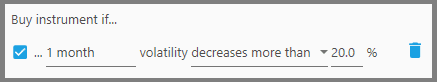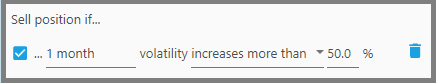This filter calculates the change of the instrument's volatility, whether the volatility is increasing or decreasing during the past certain period.

For example, if a Position's volatility increased more than 30% (for the past 3 months), then the strategy will exit out of that Position as it's becoming much riskier. In other words, the Position is more likely to maintain the implied higher volatility than you can tolerate.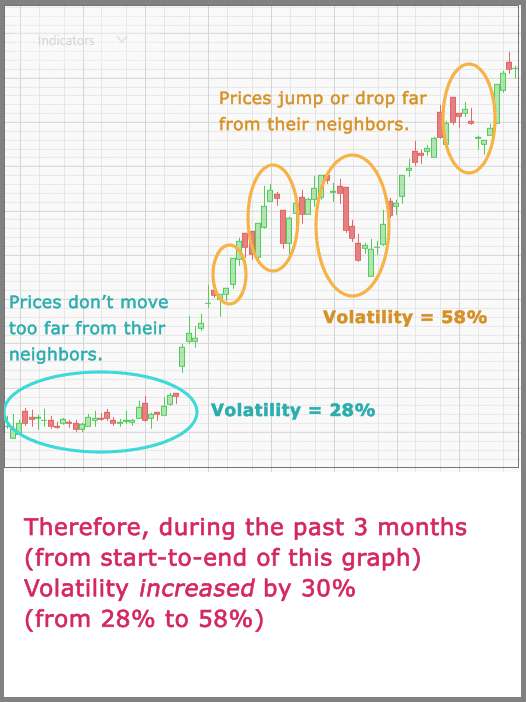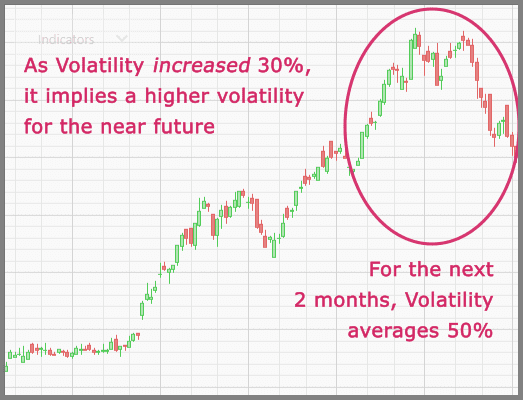Or the reverse (as a Buy Filter): if an instrument's volatility decreases more than 20% (for the whole past month), then the instrument is likely to maintain the implied lower volatility, thus a more stable trading when you enter its Position.

If an instrument's volatility doesn't change much during the specified period (either flat high volatility or flat low volatility), then its volatility percentage change will be near 0%.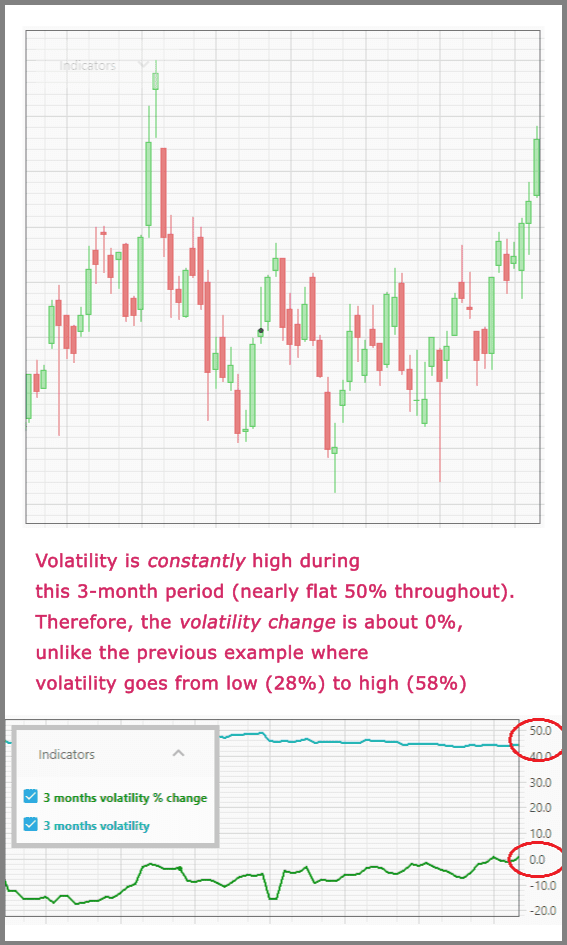1.  The first parameter defines how many days or months to look for the volatility change. The longer the period, the more accurate the signal is; that is, the implied volatility is correct.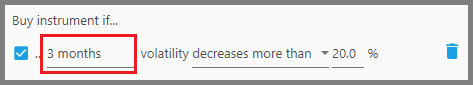2.  The second parameter defines whether the volatility must “Increase more than” or “Decrease more than” the specified percentage change. “Increase more than” implies a more volatile instrument, whereas “Decrease more than” implies a more stable instrument.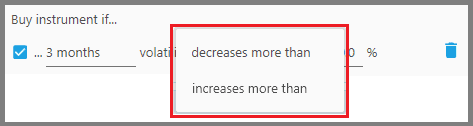Do understand that you don't want a perfectly stable instrument as you'll gain nothing (even though you lose nothing as well), as riskier instruments also carry with them a potentially higher return (despite potentially higher losses).

3.  The third parameter defines the threshold percentage change in volatility. Generally, a value of 20% is useful for generating correct signals.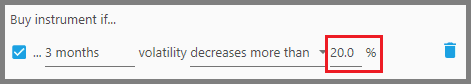#### Note:

Once you applied this filter, two indicators appear below the Price Chart. One indicator is for the instrument's volatility, while the other is for the percentage change of that volatility during the specified period.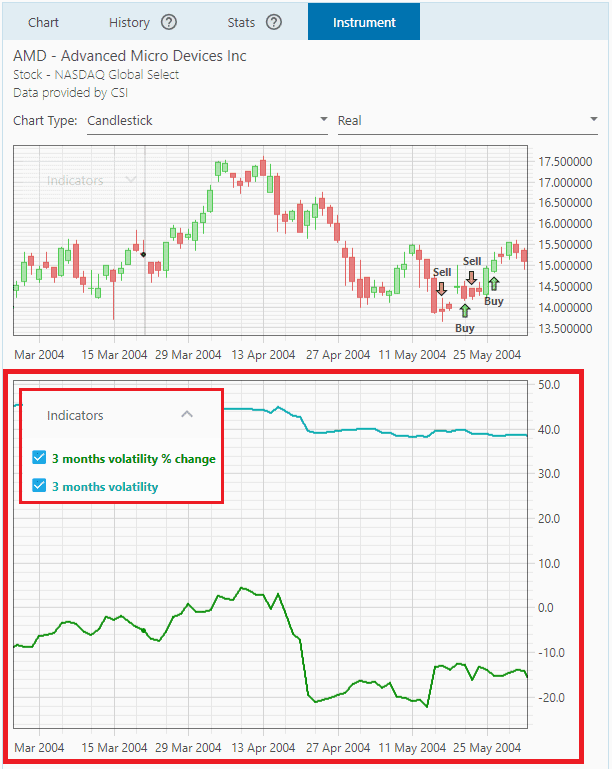Keep in mind, the percentage change may go in the negative values; that means a decrease in volatility (an overall decrease from the start of the period to the end). Remember that each point in the indicator is the reading from that point to the start of the specified period (e.g 3 months).